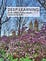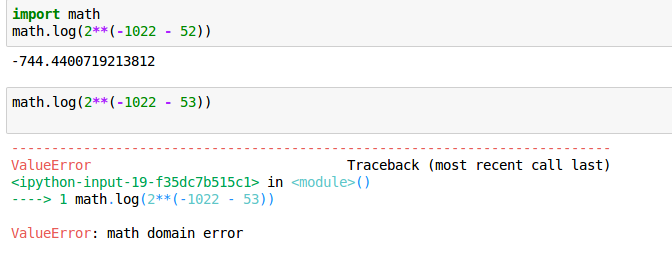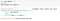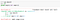# Deep Learning Book Notes: Numerical Computation Part 1

A few days back, I started reading the Deep Learning Book by Ian Goodfellow, Yoshua Bengio and Aaron Courville. Although, I haven’t read it completely, so far it has been an amazing read (I know it’s implicit!). I decided to do a series of blog posts on the basic/advanced topics I haven’t extensively gone through before. The aim of these blog articles is to serve as notes, both for me and my readers.

The first chapter I will be writing about is Chapter 4, Numerical Computation. The reason I chose this is because I learnt a lot of new stuff through this chapter, and it helped me to build a deeper intuition about the various topics covered in it!

# Starting with it!Because the post is incomplete without the glorious cover page!

## Underflow:

Numbers near 0 are rounded off to 0.

## Subsequent problems which arise:

Extremely small numbers when passed to logarithmic functions or when divided by raise exceptions.

## An example in Python:Underflow in Python

Check this StackOverflow Link for more!

## Overflow:

Numbers with large magnitudes are approximated to +∞ or -∞. Further arithmetic changes it to not-a-number.

# An example of Softmax Function:

Function Expression:

Application:

Used to predict probabilities associated with Multinoulli Probabilities.

## Case 1: c is a large negative numberAn example in Python

## Case 2: c is a large positive numberAn example in Python

## How to solve it?

Result: Adding or subtracting a scalar from input vector doesn’t change the value of softmax function.

Proof:

Using this result: Subtract maximum input feature value from all the rows.

1. The largest argument in exp will be 0, ruling out the possibility of overﬂow.
2. At least one term in the denominator has a value of 1, which rules out the possibility of underﬂow in the denominator leading to a division by zero.

An example:

1. Defining Softmax Function in Python

2. Initial Run

3. Rescaling x

4. Executing Softmax function after rescaling

Note: Underﬂow in the numerator can still cause the expression as a whole to evaluate to zero. If passing the results of softmax to another function, like log, calculate log softmax(x) in a numerically stable way, using the same trick established in calculating softmax.

## Topic 2: Poor Conditioning

Conditioning: How rapidly a function changes with respect to small change in its input.

Finding the Condition Number:

1. For a matrix function f(x) = A^(-1)x.

Here A is a nxn real-valued matrix, having an eigenvalue decomposition

This is the ratio of the magnitude of the largest and smallest eigenvalue.

Inversion is sensitive to error in input when this ratio large.

Here, sensitivity is an intrinsic property of the matrix, not the result of rounding error during matrix inversion.

With poor conditioning, pre-existing errors are amplified.

Note: The following part isn’t present in the Deep Learning Book (at least in the part I have read, yet!), but being a Mathematics enthusiast, I looked it up!

## 2. For Non-Linear single variable functions (Extra Stuff, can skip!)

Consider a function f(x). For small change △x in x,

Relative change in x = [(x+△x) -x]/x

Relative change in f(x) = [f(x+△x) -f(x)]/f(x)

ConditionNumber = Relative change in f(x) / Relative change in x

If f(x) is differentiable:

## An interesting example:

Consider the following functions:

At the first glance, it might appear that the function 1 is changing faster than function 2 with change in input, however:

## Why so?

Because we need to study the behavior of the function with infinitely small change in input!

## 3. For Non-Linear multi-variable functions (Extra Stuff, can skip! At the end of Part 2)

With this, I will be concluding the part 1 of the notes on Numerical Computation. In the next part, I will be move on to Optimization Techniques, as in the Deep Learning Book.

If you find any errors or typos or have general suggestions on how I can improve, please do comment below and I will work on it.

This was my first technical article. Looking forward to your feedback!

# References

1. Goodfellow, I., Bengio, Y., & Courville, A. (2016). Deep learning. MIT press.
2. https://github.com/roatienza/Deep-Learning-Experiments
3. https://en.wikipedia.org/wiki/Condition_number#One_variable

The logic in Mathematics. The creativity in Art. The beauty in Code.

## More from Shubhi Sareen

The logic in Mathematics. The creativity in Art. The beauty in Code.